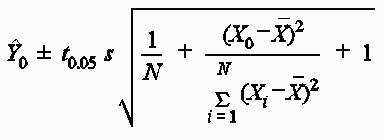### Clinic 1

Let's first check your slope and intercept estimates for your least squares line for clinic 1. Wrong answers here indicate that
2. you didn't add the new rows correctly, or
3. you typed in the clinic 1 data incorrectly.

For this answer checker, and for the write-up you hand in, round off the slope and intercept to the nearest integer (whole number). This applies to all three clinics.

What is the slope of your least squares line for clinic 1?

What is the intercept of your least squares line for clinic 1?

If those are both OK, let's test the goodness of fit statistics. Problems here indicate errors in the right half of your spreadsheet.

Is your R-squared between 0.0 and 1.0?

For the next two questions, your answer should have a decimal point and at least two numbers after the decimal point.

To test the hypothesis that the true slope is 0, you compare your t value with a critical value that you find in the proper column and row in the t table. What is that critical value?

What is your prediction, based on the least squares line, for clinic 1 for the next month, month=12?

Round off your prediction to the nearest integer. This applies to all three clinics.

If everything is correct to this point, your spreadsheet is good. All you have to do for Clinic 2 is to type or paste its visit data on top of the data for Clinic 1. The spreadsheet will recalculate, and you will have your numbers for Clinic 2.

### Clinic 2

Let's check the results of your least squares regression for clinic 2:
Type the results requested for clinic 2 in the small boxes. After typing, press Enter while you're in each box.

### Clinic 3

For Clinic 3, type or paste its visit data on top of the data for Clinic 2. The spreadsheet will again recalculate, and you will have your numbers for Clinic 3.

Let's check the results of your least squares regression for clinic 3:
Type the results requested for clinic 3 in the small boxes. After typing, press Enter while you're in each box.

### Prediction confidence interval Scroll down for help with this

What is the upper end of your prediction confidence interval?

What is the lower end of your confidence interval?

If you're having trouble with the prediction confidence interval, try these ideas:
• The upper end of the confidence interval is your predicted value plus the result of a big calculation. The lower end of the confidence interval is your predicted value minus that same result.
• The calculation of what to add and subtract from the predicted value are three things multiplied together:
• the appropriate t value from the table (not the t value from your spreadsheet)
• s, the regression's standard error
• a big square root
• The big square root has under it:
• 1/N plus
• a messy fraction plus
• 1
• The messy fraction's
• numerator is the X value for which you are predicting, 12 in this case, minus the mean of the X's. You square that difference.
• denominator is the sum of squared X deviations, which is in your spreadsheet.
• You are much better off doing this calculation in a spreadsheet, rather than by hand. If you calculate by hand, you can make a mistake without realizing it. In a apreadsheet, you can see what you did.
• In your spreadsheet, you don't need to cram all of the big formula into one cell. Instead, use several cells. For example, you can put the individual parts of the expression under the square root in three separate cells, then add them up in a fourth cell, and take the square root in a fifth cell. Then use another cell to multiply by the other pieces.

A good way to do that is to take advantage of the spreadsheet's two-dimensional layout to use spatial reasoning. Lay out the elements of the equation in the same relative positions as in the formula itself.

Here is the formula:Here is a corresponding spreadsheet layout:You can put this in the same spreadsheet page, like this, or you can put it in a separate tab.
Fill in the cells I have shaded green with cell references or formulas. (1/N and [X0 - Xavg]² are the only formulas you have to create in the green-shaded cells.) Then create formulas for the purple cells.

This kind of layout helps you see and fix errors, and it would help you remember what you did if you came back to this spreadsheet in six months.

Thanks for using the answer checker!